# Solving Linear Regression in Python

Linear regression is a common method to model the relationship between a dependent variable and one or more independent variables. Linear models are developed using the parameters which are estimated from the data. Linear regression is useful in prediction and forecasting where a predictive model is fit to an observed data set of values to determine the response. Linear regression models are often fitted using the least-squares approach where the goal is to minimize the error.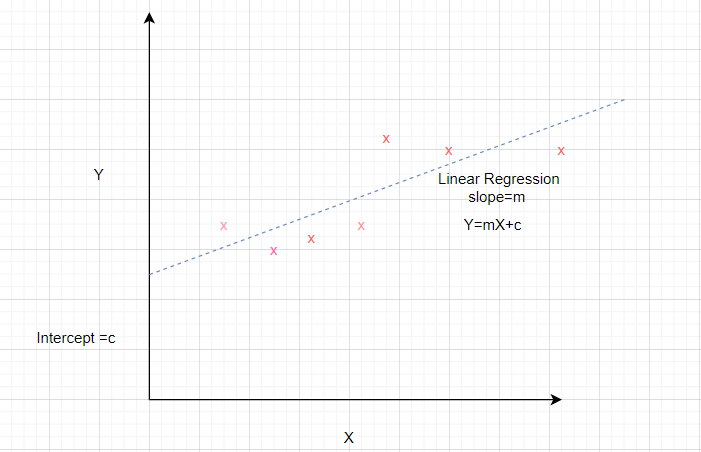Consider a dataset where the independent attribute is represented by x and the dependent attribute is represented by y.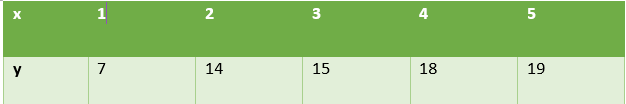It is known that the equation of a straight line is y = mx + b where m is the slope and b is the intercept.
In order to prepare a simple regression model of the given dataset, we need to calculate the slope and intercept of the line which best fits the data points.

How to calculate slope and intercept?

Mathematical formula to calculate slope and intercept are given below

```Slope = Sxy/Sxx
where Sxy and Sxx are sample covariance and sample variance respectively.

Intercept = ymean – slope* xmean```

Let us use these relations to determine the linear regression for the above dataset. For this we calculate the xmean, ymean, Sxy, Sxx as shown in the table.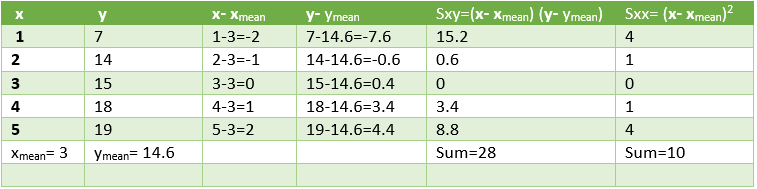As per the above formulae,
Slope = 28/10 = 2.8
Intercept = 14.6 – 2.8 * 3 = 6.2

Therefore,

`The desired equation of the regression model is y = 2.8 x + 6.2`

We shall use these values to predict the values of y for the given values of x. The performance of the model can be analyzed by calculating the root mean square error and R2 value.

Calculations are shown below.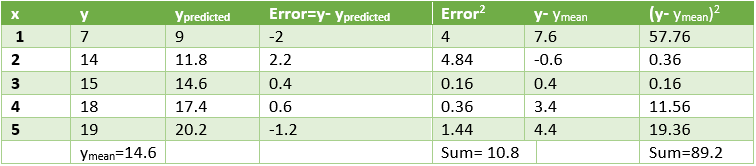Squared Error=10.8 which means that mean squared error =3.28
Coefficient of Determination (R2) = 1- 10.8 / 89.2 = 0.878

``` Low value of error and high value of R2 signify that the
linear regression fits data well```

Let us see the Python Implementation of linear regression for this dataset.

Code 1: Import all the necessary Libraries.

 `import` `numpy as np ` `import` `matplotlib.pyplot as plt ` ` `  `from` `sklearn.linear_model ``import` `LinearRegression ` `from` `sklearn.metrics ``import` `mean_squared_error, r2_score ` `import` `statsmodels.api as sm `

Code 2: Generate the data. Calculate xmean, ymean, Sxx, Sxy to find the value of slope and intercept of regression line.

 `x ``=` `np.array([``1``,``2``,``3``,``4``,``5``])  ` `y ``=` `np.array([``7``,``14``,``15``,``18``,``19``]) ` `n ``=` `np.size(x) ` ` `  `x_mean ``=` `np.mean(x) ` `y_mean ``=` `np.mean(y) ` `x_mean,y_mean ` ` `  `Sxy ``=` `np.``sum``(x``*``y)``-` `n``*``x_mean``*``y_mean ` `Sxx ``=` `np.``sum``(x``*``x)``-``n``*``x_mean``*``x_mean ` ` `  `b1 ``=` `Sxy``/``Sxx ` `b0 ``=` `y_mean``-``b1``*``x_mean ` `print``(``'slope b1 is'``, b1) ` `print``(``'intercept b0 is'``, b0) ` ` `  `plt.scatter(x,y) ` `plt.xlabel(``'Independent variable X'``) ` `plt.ylabel(``'Dependent variable y'``) `

Output:

```slope b1 is 2.8
intercept b0 is 6.200000000000001```

Code 3: Plot the given data points and fit the regression line.

 `y_pred ``=` `b1 ``*` `x ``+` `b0 ` ` `  `plt.scatter(x, y, color ``=` `'red'``) ` `plt.plot(x, y_pred, color ``=` `'green'``) ` `plt.xlabel(``'X'``) ` `plt.ylabel(``'y'``) `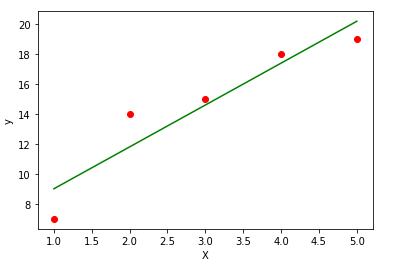Code 4: Analyze the performance of the model by calculating mean squared error and R2

 `error ``=` `y ``-` `y_pred ` `se ``=` `np.``sum``(error``*``*``2``) ` `print``(``'squared error is'``, se) ` ` `  `mse ``=` `se``/``n  ` `print``(``'mean squared error is'``, mse) ` ` `  `rmse ``=` `np.sqrt(mse) ` `print``(``'root mean square error is'``, rmse) ` ` `  `SSt ``=` `np.``sum``((y ``-` `y_mean)``*``*``2``) ` `R2 ``=` `1``-` `(se``/``SSt) ` `print``(``'R square is'``, R2) `

Output:

```squared error is 10.800000000000004
mean squared error is 2.160000000000001
root mean square error is 1.4696938456699071
R square is 0.8789237668161435```

Code 5: Use scikit library to confirm the above steps.

 `x ``=` `x.reshape(``-``1``,``1``) ` `regression_model ``=` `LinearRegression() ` ` `  `# Fit the data(train the model) ` `regression_model.fit(x, y) ` ` `  `# Predict ` `y_predicted ``=` `regression_model.predict(x) ` ` `  `# model evaluation ` `mse``=``mean_squared_error(y,y_predicted) ` ` `  `rmse ``=` `np.sqrt(mean_squared_error(y, y_predicted)) ` `r2 ``=` `r2_score(y, y_predicted) ` ` `  `# printing values ` `print``(``'Slope:'` `,regression_model.coef_) ` `print``(``'Intercept:'``, regression_model.intercept_) ` `print``(``'MSE:'``,mse) ` `print``(``'Root mean squared error: '``, rmse) ` `print``(``'R2 score: '``, r2) `

Output:

```Slope: [2.8]
Intercept: 6.199999999999999
MSE: 2.160000000000001
Root mean squared error:  1.4696938456699071
R2 score:  0.8789237668161435```

Conclusion: This article helps to understand the mathematics behind simple regression and implement the same using Python.

My Personal Notes arrow_drop_upCheck out this Author's contributed articles.

If you like GeeksforGeeks and would like to contribute, you can also write an article using contribute.geeksforgeeks.org or mail your article to contribute@geeksforgeeks.org. See your article appearing on the GeeksforGeeks main page and help other Geeks.

Please Improve this article if you find anything incorrect by clicking on the "Improve Article" button below.